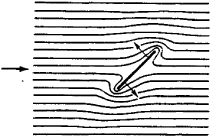# Rayleigh Disk

Also found in: Dictionary, Thesaurus.

## Rayleigh disk

[′rā·lē ‚disk]
(acoustics)
An acoustic radiometer, used to measure particle velocity, consisting of a thin disk set at an angle of 45° to a sound beam; the particle velocity is calculated from the resulting torque on the disk.

## Rayleigh Disk

(named for the English physicist J. W. Rayleigh), an instrument used to measure the intensity of sound and the vibratory velocity of particles in a sound wave. The disk is a thin circular plate made of mica or metal that is suspended on a thin filament (usually a quartz fiber). It is usually placed at a 45° angle to the direction in which the particles of the medium are vibrating (the position of maximum sensitivity). During the propagation of sound waves the disk tends to move into a position perpendicular to the direction of the vibrations. This is explained by Bernoulli’s law, which states that for a flow around the plate, the pressure is greater on the side of the disk where the velocity is lower (see Figure 1).Figure 1. Flow around a Rayleigh disk (the disk is at an angle of 45° to the flow; the forces of pressure are shown by the arrows)

The pressure forces generate a torque that is counterbalanced by the elasticity of the filament. The disk will position itself at an angle to the direction of flow that is greater than 45°. The intensity of the sound can be determined from the angle of rotation of the Rayleigh disk. In a constant flow the angle of rotation of the disk is proportional to the square of the velocity or, in the presence of sound vibrations, to the square of the amplitude of velocity. The angle of rotation does not depend on frequency.

In the USSR the Rayleigh disk has been adopted as a standard device for measuring sound pressure in a resonant pipe with standing waves. The disk is used for sound measurements not only in air and other gases but also in water.

### REFERENCE

Beranek, L. Akusticheskie izmereniia. Moscow, 1952. (Translated from English.)
Site: Follow: Share:
Open / Close